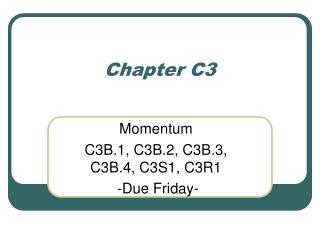DownloadDownload PresentationChapter C3

# Chapter C3

Download Presentation## Chapter C3

- - - - - - - - - - - - - - - - - - - - - - - - - - - E N D - - - - - - - - - - - - - - - - - - - - - - - - - - -
##### Presentation Transcript

1. Chapter C3 Momentum C3B.1, C3B.2, C3B.3, C3B.4, C3S1, C3R1 -Due Friday-

2. Results of C1-C2 test

3. Our plan for the future • Do chapters C3, C4 and C5 and have a test in about two weeks. • Lab Thursday will be a day to complete the velocity lab, especially the discussion part. • Next Tuesday we will begin a 3 lab sequence.

4. Velocity • Velocity is the change in position of the object divided by the time it takes the change to take place. The “≡” symbol means definition. The velocity is the time rate of change of the position of an object. Velocity is a vector. The derivative of a vector (position in this case) is also a vector.

5. Velocity dt is a very short time

6. Momentum • Momentum is mass times the velocity • In the absence of outside forces the total momentum of a system of particles remains unchanged. • During interactions between objects in an isolated system, momentum is transferred from one object to another but the total momentum of the system remains unchanged.

7. Force • The rate of change of the momentum of a particle is a measure of the force exerted on that particle. • dp is the change in momentum in the time dt caused by the interaction A. • dt is small enough so that F is constant during the interval. • This type of force is called an Impulse.

8. All interactions contribute to momentum change is the momentum change due to interaction A, etc. is the total momentum change is that due to all the interactions. When you see the word “interaction”, think “force”.

9. Force • The unit of force is the newton. • 1 N= 1kg·m/s2 6 q

10. The difference between mass and weight • Mass is the relationship between momentum and velocity • Weight is defined by Where is the gravitational field vector (gravitational force) at that point. g=mag( ) = 9.8m/s2 (on the surface of the Earth)

11. Mass and weight • It is important to note that mass and weight are very different. • Mass is an inherent property of the object • The mass of an object would remain unchanged if it were in space or on another planet • Mass is not a force! • Weight depends on the force of gravity, in fact weight is a force. • This will vary as the gravitational field changes. • As is true in space or on the moon, etc. 1 q

12. Ways of thinking about momentum conservation • The tap model applied to a projectile flying through the air. You can consider the interaction to be a series of “taps”.

13. Ways of thinking about momentum conservation • The reservoir model applied to a projectile flying through the air. You can consider the each component of the momentum to be a reservoir that is emptied or filled by the interactions. Note that in this example the momentum in the y direction is zero and that in x direction is constant (does not change). Momentum in the z direction is changed by gravity

14. Our next test will cover three chapters: C3, C4 and C5. • This text will be about September 22 – see on-line schedule • The single greatest factor in being successful in this class is your effort on the problems! • Tomorrow we will finish and hand in the vector lab. If you are already finished with this lab, work on problems during the lab time.

15. Some examples of momentum • Case 1 – The ideal collision of objects of equal mass (m), one at rest the other moving with an initial velocity of v0. After the collision, the first object is at rest. What impulse did the second car receive from the first? v0 mv0 m m mv0 What is the momentum before the collision? mv0 What must be the momentum after the collision? What must the velocity of the second object be? v0

16. Some examples of momentum • Case 2 – the ideal collision of an object of mass (m) with an initial velocity of v0 with an object of mass (2m), one at rest the other moving. After the collision, the 2m object has a velocity of ⅔v0. v0 m m m mv0 What is the momentum before the collision? mv0 What must be the momentum after the collision? What must the velocity of the first object be after the collision? -⅓v0